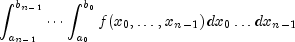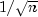JMSLTM Numerical Library 7.2.0
com.imsl.math

• All Implemented Interfaces:
Serializable, Cloneable

```public class HyperRectangleQuadrature
extends Object
implements Serializable, Cloneable```
HyperRectangleQuadrature integrates a function over a hypercube. This class is used to evaluate integrals of the form:Integration of functions over hypercubes by Monte Carlo, in which the integral is evaluated as the value of the function averaged over a sequence of randomly chosen points. Under mild assumptions on the function, this method will converge like, where n is the number of points at which the function is evaluated.

It is possible to improve on the performance of Monte Carlo by carefully choosing the points at which the function is to be evaluated. Randomly distributed points tend to be non-uniformly distributed. The alternative to a sequence of random points is a low-discrepancy sequence. A low-discrepancy sequence is one that is highly uniform.

This function is based on the low-discrepancy Faure sequence as computed by `FaureSequence`.

Example, Serialized Form
• ### Nested Class Summary

Nested Classes
Modifier and Type Class and Description
`static interface ` `HyperRectangleQuadrature.Function`
Public interface function for the HyperRectangleQuadrature class.
• ### Constructor Summary

Constructors
Constructor and Description
`HyperRectangleQuadrature(int dim)`
`HyperRectangleQuadrature(RandomSequence sequence)`
• ### Method Summary

Methods
Modifier and Type Method and Description
`double` `eval(HyperRectangleQuadrature.Function objectF)`
Returns the value of the integral over the unit cube.
`double` ```eval(HyperRectangleQuadrature.Function objectF, double[] a, double[] b)```
Returns the value of the integral over a cube.
`double` `getErrorEstimate()`
Returns an estimate of the relative error in the computed result.
`void` `setAbsoluteError(double errorAbsolute)`
Sets the absolute error tolerance.
`void` `setRelativeError(double errorRelative)`
Sets the relative error tolerance.
• ### Methods inherited from class java.lang.Object

`clone, equals, finalize, getClass, hashCode, notify, notifyAll, toString, wait, wait, wait`
• ### Constructor Detail

`public HyperRectangleQuadrature(int dim)`

`public HyperRectangleQuadrature(RandomSequence sequence)`
• ### Method Detail

• #### eval

`public double eval(HyperRectangleQuadrature.Function objectF)`
Returns the value of the integral over the unit cube.
Parameters:
`objectF` - `Function` containing the function to be integrated
• #### eval

```public double eval(HyperRectangleQuadrature.Function objectF,
double[] a,
double[] b)```
Returns the value of the integral over a cube.
Parameters:
`objectF` - `Function` containing the function to be integrated
`a` - is a `double` specifying the lower limit of integration. If null all of the lower limits default to 0.
`b` - is a `double` specifying the upper limit of integration. If null all of the upper limits default to 1.
• #### getErrorEstimate

`public double getErrorEstimate()`
Returns an estimate of the relative error in the computed result.
Returns:
a `double` specifying an estimate of the relative error in the computed result
• #### setAbsoluteError

`public void setAbsoluteError(double errorAbsolute)`
Sets the absolute error tolerance.
Parameters:
`errorAbsolute` - a `double` scalar value specifying the absolute error
• #### setRelativeError

`public void setRelativeError(double errorRelative)`
Sets the relative error tolerance.
Parameters:
`errorRelative` - a `double` scalar value specifying the relative error
JMSLTM Numerical Library 7.2.0